# Plotting topographic maps of evoked data¶

Load evoked data and plot topomaps for selected time points using multiple additional options.

```# Authors: Christian Brodbeck <christianbrodbeck@nyu.edu>
#          Tal Linzen <linzen@nyu.edu>
#          Denis A. Engeman <denis.engemann@gmail.com>
#          Mikołaj Magnuski <mmagnuski@swps.edu.pl>
#          Eric Larson <larson.eric.d@gmail.com>
#

import numpy as np
import matplotlib.pyplot as plt

from mne.datasets import sample

print(__doc__)

path = sample.data_path()
fname = path + '/MEG/sample/sample_audvis-ave.fif'

# load evoked corresponding to a specific condition
# from the fif file and subtract baseline
condition = 'Left Auditory'
evoked = read_evokeds(fname, condition=condition, baseline=(None, 0))
```

Out:

```Reading /home/circleci/mne_data/MNE-sample-data/MEG/sample/sample_audvis-ave.fif ...
Read a total of 4 projection items:
PCA-v1 (1 x 102) active
PCA-v2 (1 x 102) active
PCA-v3 (1 x 102) active
Average EEG reference (1 x 60) active
Found the data of interest:
t =    -199.80 ...     499.49 ms (Left Auditory)
0 CTF compensation matrices available
nave = 55 - aspect type = 100
Projections have already been applied. Setting proj attribute to True.
Applying baseline correction (mode: mean)
```

## Basic `plot_topomap()` options¶

We plot evoked topographies using `mne.Evoked.plot_topomap()`. The first argument, `times` allows to specify time instants (in seconds!) for which topographies will be shown. We select timepoints from 50 to 150 ms with a step of 20ms and plot magnetometer data:

```times = np.arange(0.05, 0.151, 0.02)
evoked.plot_topomap(times, ch_type='mag', time_unit='s')
```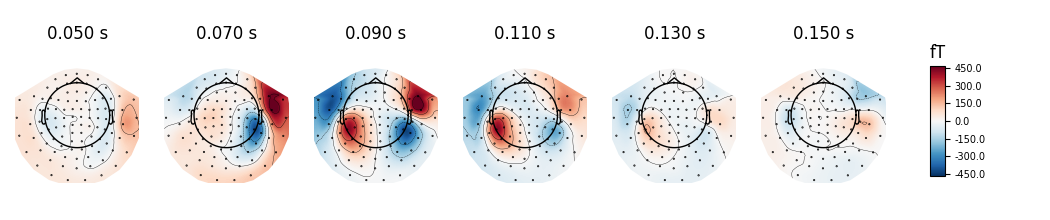Out:

```Removing projector <Projection | Average EEG reference, active : True, n_channels : 60>
```

If times is set to None at most 10 regularly spaced topographies will be shown:

```evoked.plot_topomap(ch_type='mag', time_unit='s')
```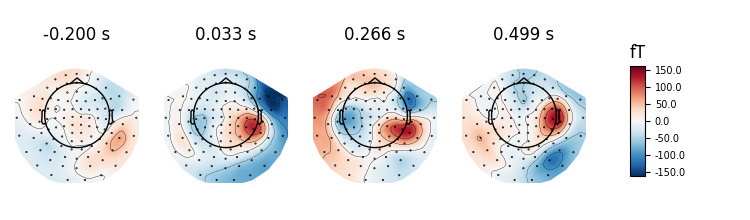Out:

```Removing projector <Projection | Average EEG reference, active : True, n_channels : 60>
```

We can use `nrows` and `ncols` parameter to create multiline plots with more timepoints.

```all_times = np.arange(-0.2, 0.5, 0.03)
evoked.plot_topomap(all_times, ch_type='mag', time_unit='s',
ncols=8, nrows='auto')
```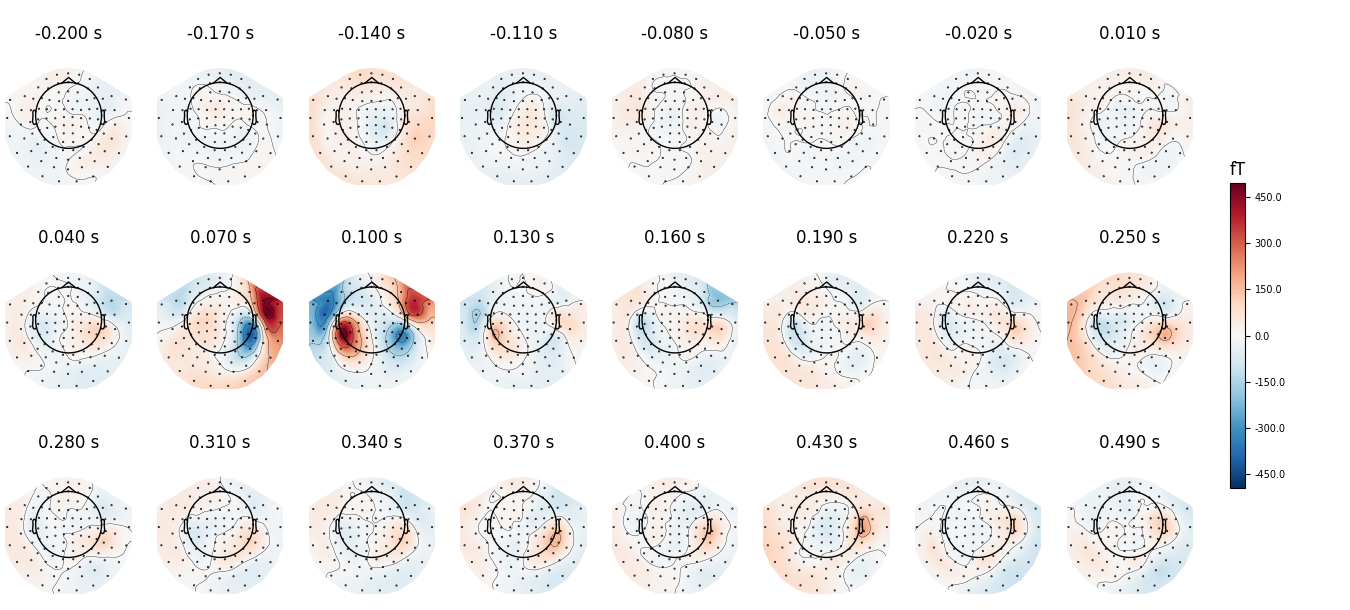Out:

```Removing projector <Projection | Average EEG reference, active : True, n_channels : 60>
```

Instead of showing topographies at specific time points we can compute averages of 50 ms bins centered on these time points to reduce the noise in the topographies:

```evoked.plot_topomap(times, ch_type='mag', average=0.05, time_unit='s')
```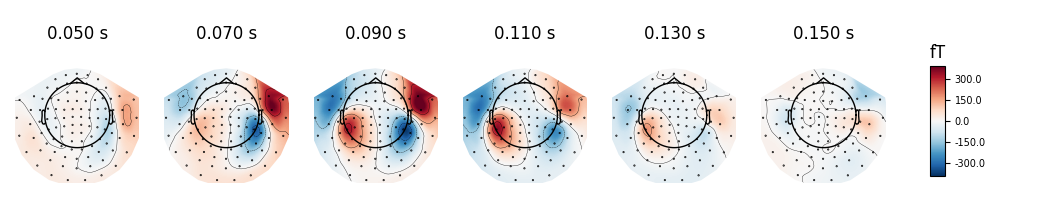Out:

```Removing projector <Projection | Average EEG reference, active : True, n_channels : 60>
```

We can plot gradiometer data (plots the RMS for each pair of gradiometers)

```evoked.plot_topomap(times, ch_type='grad', time_unit='s')
```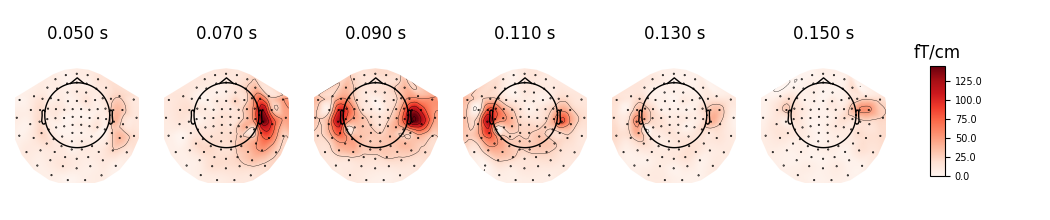Out:

```Removing projector <Projection | PCA-v1, active : True, n_channels : 102>
Removing projector <Projection | PCA-v2, active : True, n_channels : 102>
Removing projector <Projection | PCA-v3, active : True, n_channels : 102>
Removing projector <Projection | Average EEG reference, active : True, n_channels : 60>
```

## Additional `plot_topomap()` options¶

We can also use a range of various `mne.viz.plot_topomap()` arguments that control how the topography is drawn. For example:

• `cmap` - to specify the color map

• `res` - to control the resolution of the topographies (lower resolution means faster plotting)

• `outlines='skirt'` to see the topography stretched beyond the head circle

• `contours` to define how many contour lines should be plotted

```evoked.plot_topomap(times, ch_type='mag', cmap='Spectral_r', res=32,
outlines='skirt', contours=4, time_unit='s')
```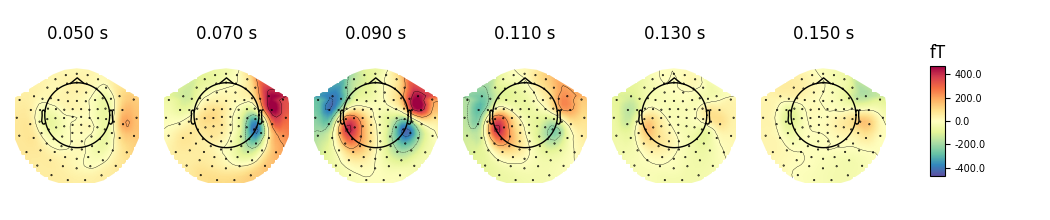Out:

```Removing projector <Projection | Average EEG reference, active : True, n_channels : 60>
```

If you look at the edges of the head circle of a single topomap you’ll see the effect of extrapolation. There are three extrapolation modes:

• `extrapolate='local'` extrapolates only to points close to the sensors.

• `extrapolate='head'` extrapolates out to the head head circle.

• `extrapolate='box'` extrapolates to a large box stretching beyond the head circle.

The default value `extrapolate='auto'` will use `'local'` for MEG sensors and `'head'` otherwise. Here we show each option:

```extrapolations = ['local', 'head', 'box']
fig, axes = plt.subplots(figsize=(7.5, 4.5), nrows=2, ncols=3)

# Here we look at EEG channels, and use a custom head sphere to get all the
# sensors to be well within the drawn head surface
for axes_row, ch_type in zip(axes, ('mag', 'eeg')):
for ax, extr in zip(axes_row, extrapolations):
evoked.plot_topomap(0.1, ch_type=ch_type, size=2, extrapolate=extr,
axes=ax, show=False, colorbar=False,
sphere=(0., 0., 0., 0.09))
ax.set_title('%s %s' % (ch_type.upper(), extr), fontsize=14)
fig.tight_layout()
```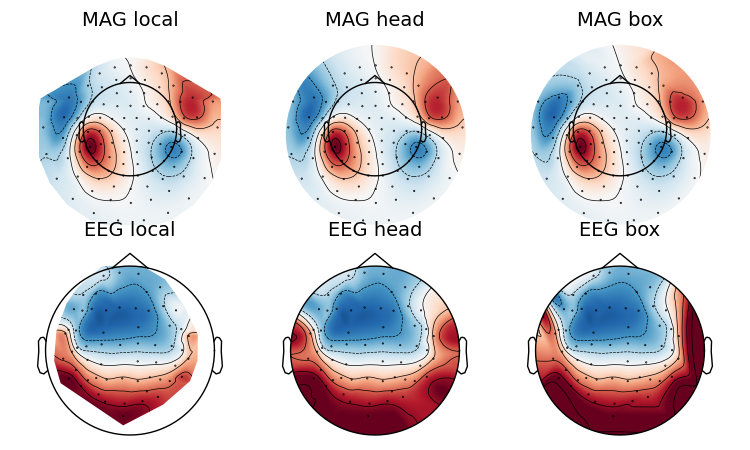Out:

```Removing projector <Projection | Average EEG reference, active : True, n_channels : 60>
Removing projector <Projection | Average EEG reference, active : True, n_channels : 60>
Removing projector <Projection | Average EEG reference, active : True, n_channels : 60>
Removing projector <Projection | PCA-v1, active : True, n_channels : 102>
Removing projector <Projection | PCA-v2, active : True, n_channels : 102>
Removing projector <Projection | PCA-v3, active : True, n_channels : 102>
Removing projector <Projection | PCA-v1, active : True, n_channels : 102>
Removing projector <Projection | PCA-v2, active : True, n_channels : 102>
Removing projector <Projection | PCA-v3, active : True, n_channels : 102>
Removing projector <Projection | PCA-v1, active : True, n_channels : 102>
Removing projector <Projection | PCA-v2, active : True, n_channels : 102>
Removing projector <Projection | PCA-v3, active : True, n_channels : 102>
```

Now we plot magnetometer data as topomap at a single time point: 100 ms post-stimulus, add channel labels, title and adjust plot margins:

```evoked.plot_topomap(0.1, ch_type='mag', show_names=True, colorbar=False,
size=6, res=128, title='Auditory response',
time_unit='s')
```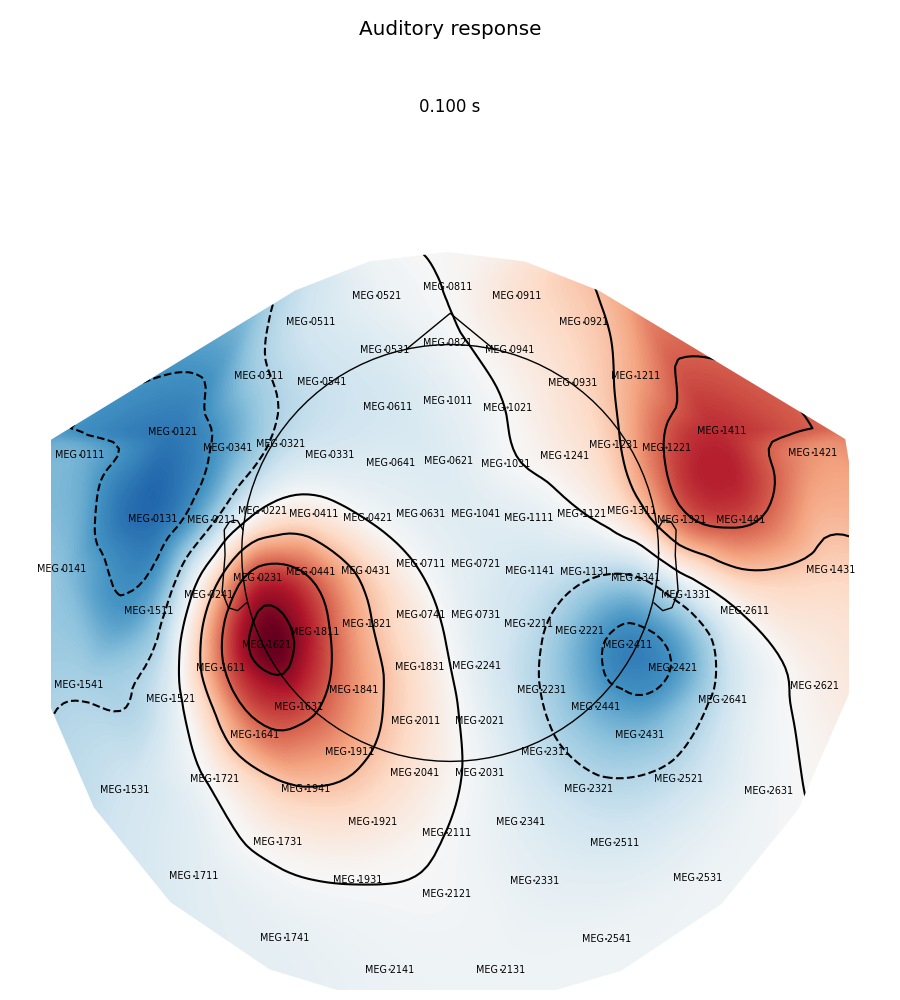Out:

```Removing projector <Projection | Average EEG reference, active : True, n_channels : 60>
```

## Animating the topomap¶

Instead of using a still image we can plot magnetometer data as an animation, which animates properly only in matplotlib interactive mode.

```times = np.arange(0.05, 0.151, 0.01)
fig, anim = evoked.animate_topomap(
times=times, ch_type='mag', frame_rate=2, time_unit='s', blit=False)
```